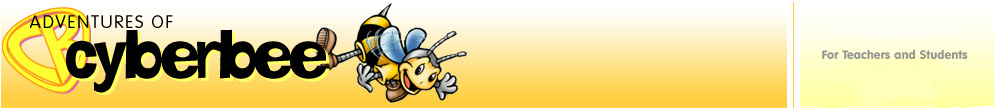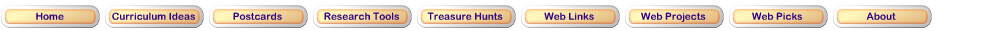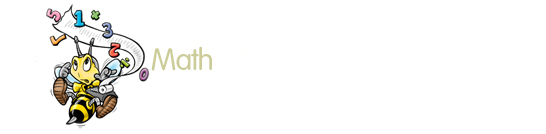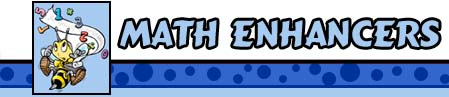NCTM Data Analysis and Probability Standard for Grades Pre-K–2 Formulate questions that can be addressed with data and collect, organize, and display relevant data to answer them Select and use appropriate statistical methods to analyze dataNCTM Measurement Standard for Grades Pre-K–2 Understand measurable attributes of objects and the units, systems, and processes of measurement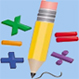Add and Subtract NCTM Number and Operations Standard for Grades Pre-K–2 Understand meanings of operations and how they relate to one anotherEven or Odd? NCTM Number and Operations Standard for Grades Pre-K–2 Understand numbers, ways of representing numbers, relationships among numbers, and number systemsNCTM Number and Operations Standard for Grades Pre-K–2 Understand numbers, ways of representing numbers, relationships among numbers, and number systems • count with understanding and recognize "how many" in sets of objects;NCTM Algebra Standard for Grades 3-5 Understand Patterns, Relations, and FunctionsNCTM Data Analysis and Probability Standard for Grades 3–5 Understand and apply basic concepts of probabilityNCTM Data Analysis and Probability Standard for Grades 3–5 Understand and apply basic concepts of probabilityNCTM Data Analysis and Probability Standard for Grades 3–5 Formulate questions that can be addressed with data and collect, organize, and display relevant data to answer themNCTM Geometry Standard for Grades 3–5 Apply transformations and use symmetry to analyze mathematical situationsNCTM Geometry Standard for Grades 3–5 Analyze characteristics and properties of two- and three-dimensional geometric shapes and develop mathematical arguments about geometric relationships Use visualization, spatial reasoning, and geometric modeling to solve problemsNCTM Measurement Standard for Grades 3–5 Understand measurable attributes of objects and the units, systems, and processes of measurementNCTM Measurement Standard for Grades 3–5 Apply appropriate techniques, tools, and formulas to determine measurementsNCTM Number and Operations Standard for Grades 3–5 Compute fluently and make reasonable estimatesNCTM Number and Operations Standard for Grades 3–5 Compute fluently and make reasonable estimatesNumber and Operations Standard for Grades 3-5 Understand numbers, ways of representing numbers, relationships among numbers, and number systemsAnalyzing Change Analyzing Change Standards Grades 6-8 Identify and describe situations with constant or varying rates of change, and compare them. Use technology to analyze change; e.g., use computer applications or graphing calculators to display and interpret rate of change.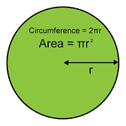Know the formulas for the area and circumference of a circle and use them to solve problems; give an informal derivation of the relationship between the circumference and area of a circle.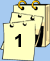Pi and Circumference of a CircleArea of a CircleReview and Problems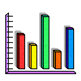Data Analysis Data Analysis Standards 6-8 Discuss and understand the correspondence between data sets and their graphical representations, especially histograms, stem-and-leaf plots, box plots, and scatterplots. Use observations about differences between two or more samples to make conjectures about the populations from which the samples were taken.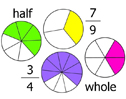Work flexibly with fractions to solve problems. Compare and order fractions efficiently and find their approximate locations on a number line.Introduction to FractionsMultiplying and Dividing FractionsFrom Fractions to DecimalsWork flexibly with fractions, decimals, and percents to solve problems.

Develop meaning for percents greater than 100 and less than 1.From Decimals to FractionsDecimals Less Than 1PerimeterAreaReview and Assessments

Solve real world and mathematical problems involving perimeters of polygons, including finding the perimeter given the side lengths, finding an unknown side length, and exhibiting rectangles with the same perimeter and different areas or with the same area and different perimeters.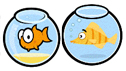Proportional Reasoning Proportional Reasoning Standards Grades 6-8 Compute unit rates associated with ratios of fractions, including ratios of lengths, areas and other quantities measured in like or different units. Recognize and represent proportional relationships between quantities. Develop, analyze, and explain methods for solving problems involving proportions, such as scaling and finding equivalent ratios..A Maths Dictionary for Kids 2008 by Jenny Eather. This is a wonderful visual dictionary with many interactive illustrations. It is highly recommended for students and teachers.Updated April 8, 2017
© 1996 - 2009 Linda C. Joseph